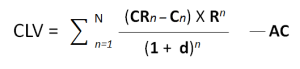# The CLV Equation

## The customer lifetime value formula in equation form

There are two articles on this website that discusses the customer lifetime value (CLV) formula as well as providing a table of numbers to follow to show how the CLV equation is executed. For most people, it is probably better to review that section or simply use the free Excel template to calculate customer lifetime value.

However, if you are more comfortable with mathematics and equations here is the formal equation for calculating customer lifetime value (CLV):### In this CLV equation:

• CR = customer revenues
• C = customer costs
• R = retention rate
• d = discount rate
• AC = acquisition rate

#### Working through the mechanics of the CLV equation:

The Greek letter sigma at the start of the equation is simply summing each calculation. The first calculation starts in year one (n = 1) and continues to add up the results until the last year under consideration (N).

CR – C is revenues less costs for each year (n) as equates to the customer’s profit contribution.

This profit contribution is then multiplied by the retention rate, which is reducing over time as customers leave year after year. This reducing customer retention rates over time acts as a probability estimate of the likelihood of the firm receiving the customer’s revenue in the future (in the calculation).

All of this is divided by increasing discount rate underneath the equation.

As suggested by the sigma sign, each year is calculated and then all results are added together. From the total customer profit contribution the initial acquisition cost is deducted to determine the final customer lifetime value (CLV).

###### Related topics

Simple CLV formula

The main CLV formula

An example of calculating customer lifetime value

The free Excel templates to calculate the CLV equation